# Modulus Function(Absolute Value function)

## Modulus Function

Modulus Function is defined as the real valued function $f : R \rightarrow R$ , y = |x| for each $x \in R$
For each non-negative value of x, f(x) is equal to x. But for negative values of x, the value of f(x) is the negative of the value of x
$f(x) = |x|= \begin{cases} x & \text{ if } x \geq 0 \\ -x & \text{ if } x < 0 \end{cases}$

It is also called the absolute value function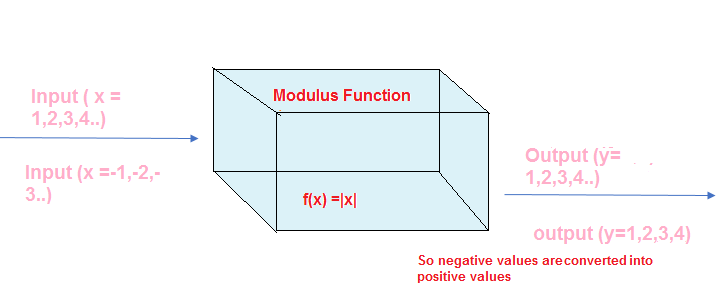Example
|-3| =3
|-2|=2
|2|=2

We know that all the real number can be plotted on the real number line. The absolute value function is used to measure the distance between two numbers on the number line.. Thus, the distance between x and 0 is |x - 0| = |x|. So |x| represent the distance of the number from the origin.Similarly the distance between x and y is |x - y|. Thus, the distance from -3 to -1 is |-3 - (-1)| = |-3 + 1| = |2| = 2, and the distance from -3 to 5 is |-3 - 5| = |-8| = 8

## Domain and Range of the Modulus Function(absolute value function)

For $f : R \rightarrow R , y = f(x) = |x|$ for each $x \in R$

Domain = R
Range = $R^+ ={x \in R : x \geq 0}$

## Graph of the Modulus Function

The graph of the modulus function is shown below. It coincide with the graph of Identity Function $y=x$ for $x \geq 0$ and for x< 0, it is a graph of Linear Function $y=-x$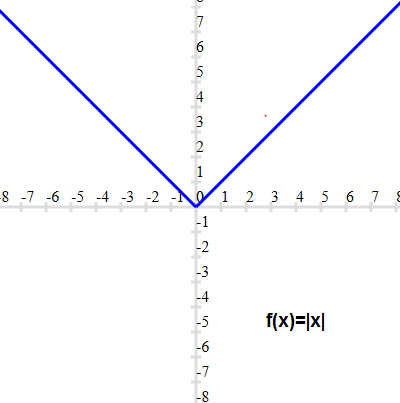Here at x=0 ,the graph is turning upward. So x=0 is the turning point in this graph

## Properties of the Modulus Function

The modulus function has the following properties

1. For any real number x , we have
$\sqrt {x^2} =|x|$

2. ||x||= |x|

3. if a and b are positive real numbers
a. $x^2 \leq a^2 \Leftrightarrow |x| \leq a \Leftrightarrow -a \leq x \leq a$
b. $x^2 \geq a^2 \Leftrightarrow |x| \geq a \Leftrightarrow x \leq -a \; or x \geq a$
c. $x^2 < a^2 \Leftrightarrow |x| < a \Leftrightarrow -a < x < a$
d. $x^2 > a^2 \Leftrightarrow |x| > a \Leftrightarrow x < -a \; or x > a$
e. $a^2 \leq x^2 \leq b^2 \Leftrightarrow a \leq |x| \leq b \Leftrightarrow x \in [-b,-a] \cup [a,b]$
f $a^2 < x^2 < b^2 \Leftrightarrow a < |x| < b \Leftrightarrow x \in (-b,-a) \cup (a,b)$

4. if a is negative
a. |x| \geq a , x \in R$b. |x| \leq a , x =\phi$

3. For real number x and y
a. |xy|=|x||y|
b. $|\frac {x}{y}| =\frac {|x|}{|y|} ,y \neq 0$
c. $|x +y | \leq |x| + |y|$
$|x -y| \leq |x| + |y|$
for $x,y \geq 0 \; or \; x,y < 0 , |x+y| =|x| + |y|$
d. $|x + y | \geq |x| - |y|$
$|x -y| \geq |x| - |y|$
for $x,y \geq 0 \; and \; |x| \geq |y| y or \; x,y < 0 \; and \; |x| \geq |y| , |x- y| =|x| - |y|$

## Solved examples of Modulus Functions(absolute value function)

1. Find the domain and range of the below function?
a. $y =|1-x|$
b. $y = 2 -|1-x|$
c. $y= \frac {2}{\sqrt {x -|x|}}$
Solution

a. $y =|1-x|$
Clearly This is defined for $x \in R$. So Domain is R
Now $|1 -x| \geq 0$ for all $x \in R$
So Range is $[0,\infty )$

b. $y = 2 -|1-x|$
Clearly This is defined for $x \in R$. So Domain is R
Now $|1-x| \geq 0$ for all $x \in R$
or
$-|1-x| \leq 0$
$2- |1-x| \leq 2$ for all $x \in R$
So Range is $(-\infty,2]$

c.$y= \frac {2}{\sqrt {x -|x|}}$
Now
$x -|x| = \begin{cases} x-x=0 & \text{ if } x \geq 0 \\ x+x=2x & \text{ if } x \leq 0 \end{cases}$

Therefore, $\frac {2}{\sqrt {x -|x|}}$ is undefined for all $x \in R$
So domain of the function is $\phi$

2. Draw the graph of the below function?
a. y=x + |x|
b. y = x -|x|
c. y =|x-1|
Solution
We need to convert this function into simple form before drawing the graph
a. y=x + |x|
This can be written as
$y= x +|x| = \begin{cases} x+x=2x & \text{ if } x \geq 0 \\ x-x=0 & \text{ if } x < 0 \end{cases}$
So, This is a linear function y =2x for $x \geq 0$ and linear function y =0 for $x < 0$
So graph will be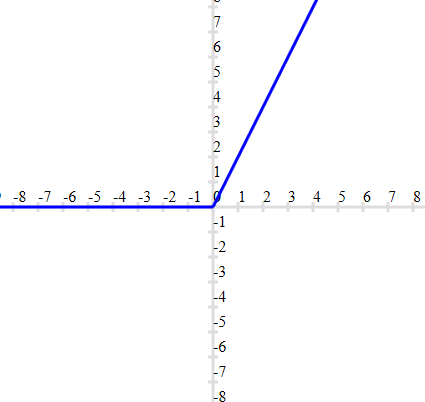b.y = x -|x|
This can be written as
$y= x -|x| = \begin{cases} x-x=0 & \text{ if } x \geq 0 \\ x+x=2x & \text{ if } x < 0 \end{cases}$
So, This is a Linear Function y =0 for $x \geq 0$ and linear function y =2x for $x < 0$
So graph will be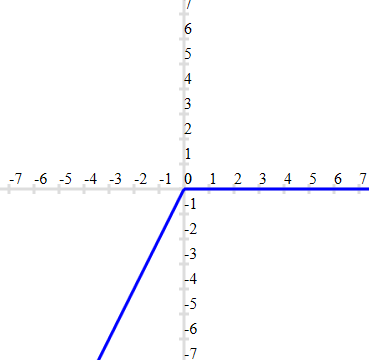c.y =|x-1|
This can be written as
$y= |x -1| = \begin{cases} x-1 & \text{ if } x \geq 1 \\ 1 -x & \text{ if } x < 1 \end{cases}$
So, This is a linear function y =x-1 for $x \geq 1$ and linear function y =1-x for $x < 1$
So graph will be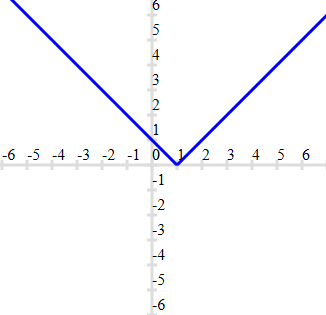Important thing to note here. Turning point of the graph is x=1 here

### Quiz Time for Absolute value function

Question 1.
The function y =|x -5|. which one of the below is incorrect
A.y(0)=5
B. y(-5)=0
C. y(-1)=6
D. y(10)=5
Question 2.
Find the range of the function $y= \frac {|x -5|}{x-5}$
A. range={1}
B. range=R
C.range={-1,1}
D. range=$[0,\infty )$
Question 3.
Find the domain of the function given in previous question
A. R -{5}
B. R
C. R -{-5}
D. $R^+$
Question 4.
Let $f(x) =|x-1| + |x-3| + |x-4|$,which one of the below is incorrect
A. f(1) =4
B. f(3.5)=3.5
C. f(5)=7
D. f(0)=7
Question 5.
The range of the function y =x|x|
A. R
B. $R^+$
C. $R^-$
D. None of these
Question 6.
The domain and range of the function f given by f (x) = 11 – |x −5| is
A. Domain = R, Range =R
B. Domain = R , Range = $(-\infty ,11]$
C. Domain = R , Range = R -{11}
D. Domain = R – {11}, Range = R